# 3. Curvilinear motion

## Introduction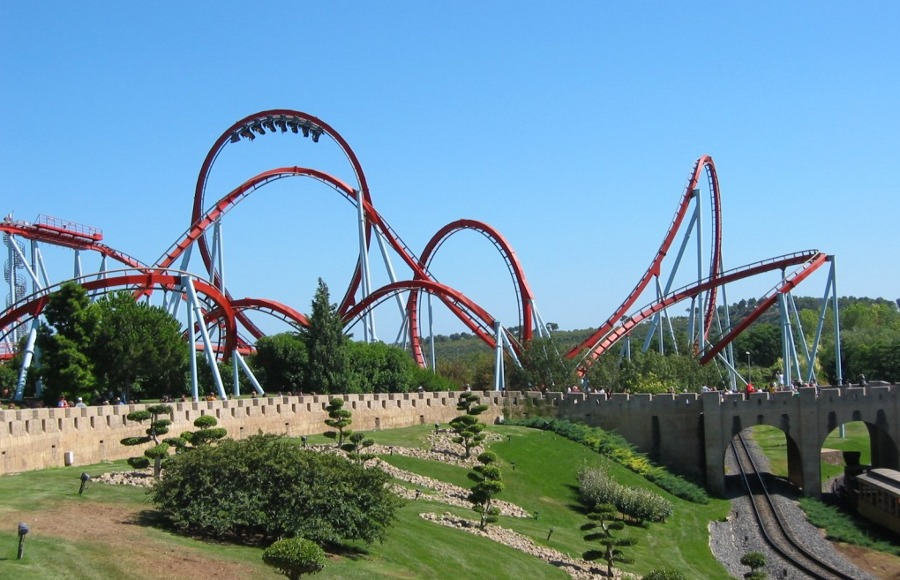The strong accelerations experienced in a roller coaster are not only due to the changes of the speed, but also to the curved trajectory. The rate of change of the speed is only one of the components of the acceleration, namely, the tangential component. The other component of the acceleration depends on the curvature of the trajectory as it is shown in this chapter.

## 3.1. Tangential unit vector

A tangential unit vector can be defined at each point of the trajectory, in the direction tangent to it and in which increases. Figure 3.1 shows the tangential unit vector in three points A, B and P of a trajectory.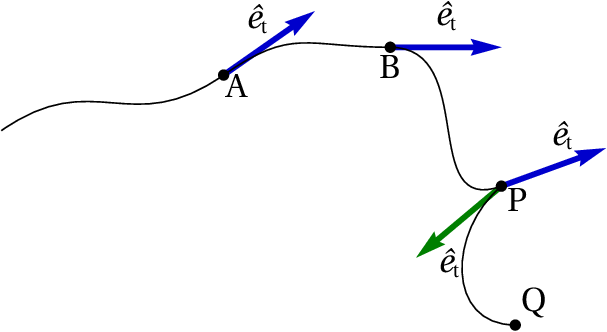Figure 3.1: Tangential unit vector ˆ e t in three points of the trajectory.

Note that there are two different tangential unit vectors at point P. One of them is tangent to the curve between B and P and the other is tangent to the curve between P and Q. The velocity vector of an object that follows that trajectory will always be in the direction of the tangential unit vector, or in the opposite direction. In points such as P, where there are two different tangential unit vectors, the velocity will necessarily be zero; the object will be momentarily at rest at that point, starting to move again in a different direction from the one it had before it stopped.

In points where the velocity is different from zero, there is always only one tangential unit vector , which determines the direction of the velocity vector. Namely, the velocity vector can be written,

(3.1)

As mentioned in Chapter 2, the velocity vector is given by the derivative of the position vector

(3.2)

Since the position vector depends on the choice of the origin of the reference frame, it does not have any relation to the tangential unit vector (see Figure 3.2). However, the displacement vector is independent of the choice of origin and therefore, equation 3.2 ensures that the velocity vector is also independent of the choice of the origin.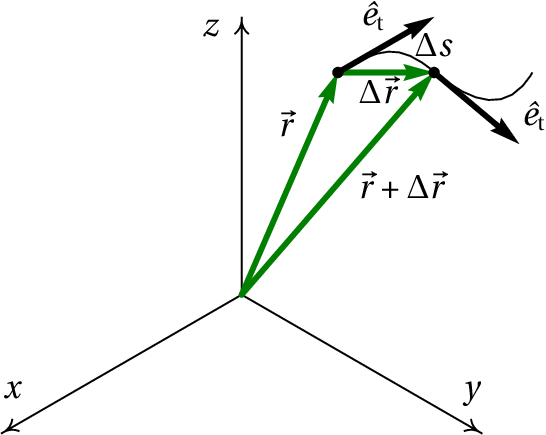Figure 3.2: Displacement vector between two positions  r and  r + Δ   r .

If Δ  is the displacement vector during a time interval (Figure 3.2), the distance traveled during that interval, , is greater than or equal to the magnitude of Δ  . While the distance traveled is measured along the trajectory, the magnitude of the displacement is measured along the straight-line segment from the initial to the final position.

The magnitude of Δ  is equal to Δ  only when the trajectory is a straight line, with a constant tangential unit vector. In the limit as Δ  approaches zero, the initial and final position are very close to each other, the direction of Δ  is approximately the same direction of the tangential unit vector and the magnitude of Δ  is approximately equal to the distance traveled ; hence, the displacement vector is approximately equal to . The derivative of the position vector is then equal to

(3.3)

And from equation 3.2 it follows that

(3.4)

In any kind of motion, the velocity is always equal to the derivative of the position along the trajectory, , with respect to time. This is why in Chapter 1 the derivative was called velocity, because it is not just the component of the velocity along the trajectory, but the velocity itself.

## 3.2. Normal unit vector

The acceleration vector is given by the derivative of the velocity vector with respect to time. Hence, the relation between the acceleration and the tangential unit vector can be found differentiating both sides of equation 3.4:

(3.5)

Note that the derivative of the tangential unit vector is not null because that vector is not necessarily the same at different instants. Figure 3.3 shows how to compute the variation of the tangential unit vector during the time interval of time shown in Figure 3.1. The tangential unit vectors at the beginning and end of the interval (points A and B) were placed in a common point and the variation of the tangential unit vector, , is the vector from the terminal point of the first vector to the terminal point of the second one.

Since the magnitude of is 1, the terminal points of the two unit vectors in Figure 3.3 are along an circular arc with radius equal to 1 and angle Δ  . If the angle is given in radians, the length of the arc is Δ  . In the limit as the time interval Δ  approaches zero, the initial an final points A and B will be very close to each other, the vector will become perpendicular to the trajectory and its magnitude will be approximately equal to the length of the circular arc, Δ  ; it is then concluded that the derivative of is

(3.6)

where is the normal unit vector, which is perpendicular to the trajectory and is the angular velocity. The expression for the acceleration vector is obtained substituting this derivative into equation 3.5:

(3.7)

In conclusion, the acceleration is a vector with components tangent and normal (perpendicular) to the trajectory. The tangential component, , is the tangential acceleration, which was introduced in Chapter 1. The normal component of the acceleration is equal to the product of the velocity and the angular velocity

(3.8)

Since the unit vectors and are perpendicular in any point of the trajectory, equation 3.7 implies that the magnitude of the acceleration, , is the length of the hypotenuse of a right triangle where the other two sides are the tangential and normal components of the acceleration; the Pythagorean theorem applied to that triangle leads to

(3.9)

The angle Δ  that the tangential unit vector rotates is also to the same angle that the normal unit vector rotates. Figure 3.4 shows the normal unit vectors in the same points shown in Figure 3.1. Note that in A there are two normal unit vectors, with opposite directions, because the trajectory curves towards the top, before point A, and it then curves toward the bottom after point A. That type of point, where the direction of the curvature changes side, is called inflection point.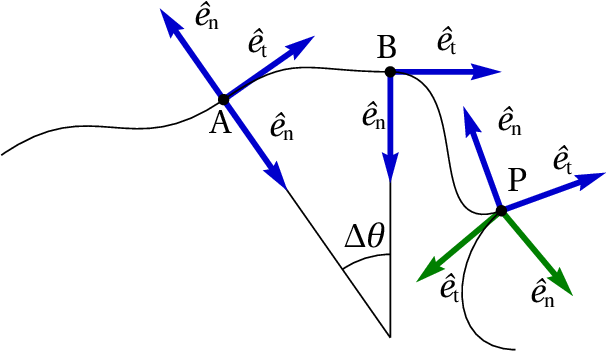Figure 3.4: Tangential and normal unit vectors in some points of the trajectory.

In point P of Figure 3.4 there are also two normal unit vectors, because as it was explained in the previous section, there are also two different tangential unit vectors. In any point the normal unit vector points in the direction where the trajectory is curving, except in the case of a rectilinear trajectory, in which there is an infinite number of normal unit vectors .

Figure 3.5 shows the normal unit vector in the initial position A (at time ) and the final position B (at time + Δ  ) during a time interval Δ  . If Δ  is very small, the directions of the two unit vectors cross at a common point C. The distances from C to the two points A and B ( e ) are different but approach to each other in the limit Δ  → 0, when point C approaches the center of curvature of the trajectory. The distance from the center of curvature to the point on the trajectory is the radius of curvature, , at that point of the trajectory.

In each point of the trajectory there is a center of curvature and a radius of curvature. Each infinitesimal displacement can be approximated by a circular arc of radius and angle ; the distance traveled is equal to the length of that circular arc, . Therefore, the angular velocity is given by

(3.10)

Namely, in each point of the trajectory the angular velocity is equal to the velocity divided by the radius of curvature at that point. That result can be used to express the normal acceleration in the following way modo seguinte

(3.11)

The normal unit vector and the normal component of the acceleration always point in the direction of the center of curvature. Hence, the normal acceleration is also called centripetal acceleration.

Note that the tangential acceleration can be either positive or negative, while the normal or centripetal acceleration is always positive, because the product is always positive ( and both increase, if the motion is in the direction of the tangential unit vector, or both decrease if the motion is in the opposite direction).

### Example 3.1

The position of a particle as a function of time is given by the following expression (SI units):

Find the expression for the radius of curvature of the trajectory as a function of time and compute the radius of curvature at the two instants = 0 and = 1.

Solution: To obtain the expression for the radius of curvature it is necessary to find the expressions for the velocity and the normal acceleration. Those two expressions can be obtained from the velocity and acceleration vectors. In Maxima those vectors can be obtained in the following way

(%i1) vector_r: [5*t, 3*t^2/2, 2*(1-t^2)]\$
(%i2) vector_v: diff (vector_r, t);
(%o2)
(%i3) vector_a: diff (vector_v, t);
(%o3)

The magnitudes of and can be found from the square root of the product of each of these vectors with itself. The scalar product of two vectors is computed in Maxima writing a dot between the two lists that represent the vectors:

(%i4) v: sqrt (vector_v.vector_v);
(%o4)
(%i5) a: sqrt (vector_a.vector_a);
(%o5)

Note that the acceleration is constant, which implies a trajectory either linear or parabolic. To find the normal component of the acceleration vector, the tangential component can be found first through the derivative

(%i6) at: diff (v, t);
(%o6)

and the normal component is found from equation 3.9:

(%i7) an: ratsimp (sqrt (a^2 - at^2));
(%o7)

The tangential and normal components of the acceleration both depend on time, in spite of the acceleration being constant; that suggests that the radius of curvature does not remain constant and, hence, the trajectory is parabolic. The expression for the radius of curvature can be obtained from equation 3.11

(%i8) R: ratsimp (v^2/an);
(%o8)

When  = 0 and  = 1 the radius of curvature is then

(%i9) subst (t=0, R);
(%o9)
(%i10) float (subst (t=1, R));
(%o10)

## 3.3. Circular motion

When the radius of curvature of the trajectory remains constant, the trajectory is a circumference and the motion is circular, as in the case shown in Figure 3.6. Only one degree of freedom is needed in order to give the position in any instant; that degree of freedom can be either the position along the circumference, , or the angle .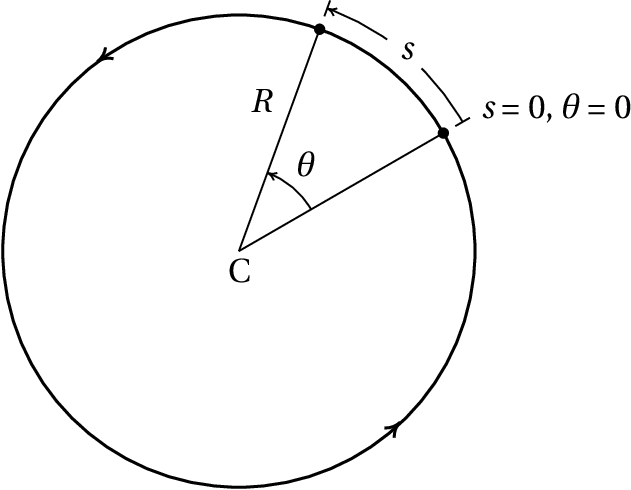Figure 3.6: Two positions in the trajectory of a circular motion.

If the angle and the position on the trajectory are measured starting from the same point and both in the same direction, the relation between them is (see Figure 3.6)

(3.12)

Since is constant, differentiating both sides of this last equation leads to

(3.13)

where is the angular velocity. Equation 3.13 is the same as equation 3.10, which was obtained here for the particular case of circular motion, where is constant, but it is a general equation valid for any other curvilinear motion. Differentiating both sides of equation 3.13 with respect to time, another expression for the tangential acceleration is found

(3.14)

where is the angular acceleration. The centripetal acceleration is given by equation 3.11, which can be written in terms of the angular velocity

(3.15)

In the particular case when the angular velocity remains constant, the linear velocity is constant too, the angular and tangential accelerations are null and the motion is called uniform circular motion. In that case, since the angular velocity is constant, its derivative can be computed dividing the angle for any interval of time by the value of that interval:

(3.16)

During an interval of time equal to the period of the circular motion, any point in the object moves one turn around the circumference and the corresponding angle is Δ   = 2  ; therefore, the period of rotation is

(3.17)

The frequency of rotation, , defined as the inverse of the period, indicates the number of turns that the point moves per unit time.

The relations among the rotation angle , the angular velocity and the angular acceleration are similar to the relations among the position on the trajectory , the velocity and the tangential acceleration

(3.18)

These are the kinematic equations for a circular motion, which can be solved using the same methods used in Chapter 1. The equations 3.12, 3.13 and 3.14 show that the kinematic variables related to translation ( , , ) are all equal to the corresponding kinematic variable for the circular motion, ( , , ), multiplied by the radius of curvature .

## 3.4. Rigid bodies kinematics

Figure 3.7 shows a moving rigid body. Point O' is the origin of a fixed external reference frame and point O is a point attached to the body, used as origin for a reference frame that moves with the body.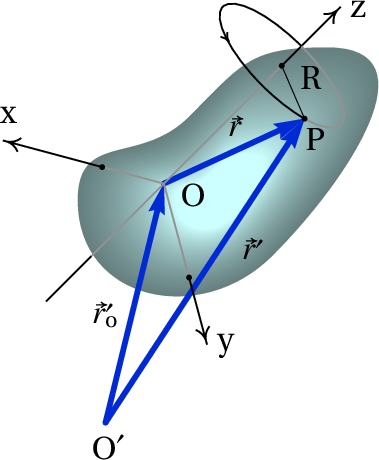Figure 3.7: Moving rigid body and reference frame O x y z attached to it.

The position vector of a point P in the rigid body is , with respect to the fixed reference frame, and with respect to the reference frame moving with the body. The relation between those two vectors is the following

(3.19)

In the reference frame O , in which point O is not moving, any feasible motion of the rigid body has a line of points, passing through O, which also remain stationary. It would be impossible to have a motion in which all the points in the rigid body move except O. The straight line of stationary points is the rotation axis of the body and in Figure 3.7 it was chosen as the axis. In different instants the rotation axis might be different, but the only motions studied in this chapter will be plane rotations, defined as the motion in which the rotation axis is always pointing in the same direction. Thus, it is assumed that the axis moves with the body but keeps always the same direction. The other two axis and , will be chosen also with a fixed direction while they move with the body. Namely, with respect to the reference frame O the motion of the rigid body is pure rotation, without translation.

Since the three unit vectors , and of the moving frame O remain constant, the relations derived in Section 2.2 are valid, so the velocity and acceleration vectors of a point P in the rigid body, with respect to the fixed frame, are equal to the velocity and acceleration vectors with respect to the moving frame, plus the velocity and acceleration vectors of point O, with respect to the fixed frame

(3.20)

The magnitude of the position vector and the angle it makes with the axis remain constant, as shown in Figure 3.7. Figure 3.8 shows the motion of point P, as seen in the moving frame, which is a circular motion on a plane parallel to the plane, with center on the axis and radius .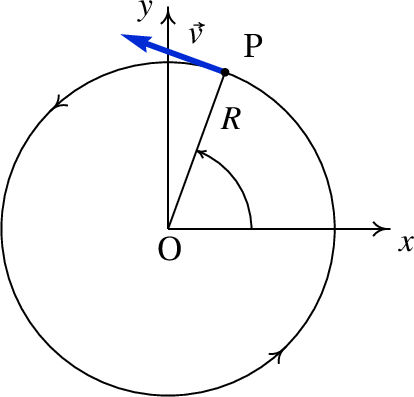Figure 3.8: Trajectory of a point in the reference frame moving with the rigid body.

In the moving frame, the velocity vector and the acceleration vector are those derived in the previous section for a circular motion; the velocity is then

(3.21)

and the tangential and normal components of the acceleration are

(3.22)

The vector expressions for the velocity and acceleration are more conveniently obtained in a system of cylindrical coordinates. Figure 3.9 shows the three cylindrical coordinates ( , , ) of point P. The plane through P and parallel to the plane intersects the axis at point Q; is the distance between O and Q and is the distance between P and Q. The angle is the angle between the projection of the line segment on plane, and the positive side of the axis.

The three perpendicular unit vectors associated to the cylindrical coordinates are , and . The unit vector is fixed, while the other two unit vectors point in different directions at different points but are always on a plane parallel to the plane. The unit vector is in the direction of the line segment , pointing away from the axis. The direction of the unit vector is tangent to the circumference that passes through P and has center in Q, in the direction in which increases.

The velocity vector has the same direction as the unit vector . Since the angular velocity is equal to the derivative of the angle with respect to time, a positive value for implies rotation in the direction in which increases and if is negative the rotation is in the opposite direction. Hence, an expression for the velocity vector is

(3.23)

The tangential component of the acceleration vector is either in the same direction of the unit vector or in the opposite direction, and the normal component of is in the opposite direction of the unit vector . It then follows that

(3.24)

## 3.5. Angular velocity vector

It is convenient to define the angular velocity vector , with the direction of the rotation axis as shown in Figure 3.10. The magnitude of the vector is the absolute value of the angular velocity, . There are two opposite directions along the rotation axis; the direction of is chosen according to the right-hand rule, namely, if the points of the rigid body that pass through the positive side of the axis move towards the positive side of the axis, the angular velocity vector is then in the direction of the axis. If those points move towards the negative side of the axis, the direction of is then opposite to the direction of the axis. Another way to use the right-hand rule to determine the direction of consists on closing the right-hand fingers keeping the thumb open and perpendicular to the other fingers; if the four closed fingers are positioned following the direction of the rotation, the thumb then points in the direction of .

The advantage of using a vector to represent the angular velocity is that the information about the rotation plane, the direction of rotation in that plane and the rotation speed is all contained within the vector . Also, equation 3.23 can then be written in vector form, independently from the coordinates system used, via the cross product

(3.25)

The cross product of two vectors and is defined as another vector (to be read as cross ), with magnitude equal to the product of the magnitudes of and and the sine of the angle between them. In particular, the magnitude of is . Figure 3.10 shows the angle between the two vectors; note that is always positive, because is between 0 and . The product is equal to , because that distance is measured on the rotation plane, which is perpendicular to . Therefore, the magnitude of is equal to , which is also the magnitude of .

The direction of is perpendicular to the plane of and , following the right-hand rule from to : if the right-hand index finger points in the direction of and the middle finger points in the direction of , then is in the direction of the thumb. Figure 3.10 shows the plane of and , which is perpendicular to the plane so the direction of is parallel to the plane, perpendicular to the plane of and and following the direction of the rotation.

The cross product is not commutative; namely, and are not equal because they have the same magnitude but opposite directions. Since the angle of a vector with itself is zero, the cross product is null. In particular, = = = 0. The cross product between two perpendicular unit vectors is another unit vector perpendicular to the plane of them. It is easy to check that = , = and = . Using this results and the distributive law for the cross product, an expression for the cross product can be obtained in terms of the Cartesian components of the two vectors

(3.26)

and this result can be written in a more compact form with a determinant:

(3.27)

Note that the shaded triangle in Figure 3.10 has base equal to and height equal to ; therefore, its area is equal to half the magnitude of cross : . In general,

The area of a triangle formed by two vectors with a common initial point is equal to half the magnitude of the cross product of those vectors.

The components of the acceleration of a point in a rigid body, relative to the reference frame moving with the body, are given by equation 3.24, which can be written using cross products

(3.28)

where is the angular acceleration vector, equal to the derivative of with respect to time. It should be remembered that this last expression is only valid for plane rotations, where the directions of the three axis of the moving frame remain constant and the differentiation of to obtain must be carried out with respect to that moving frame.

### Example 3.2

A string is attached and winded around a pulley with radius of 5 cm and a block is hanged to the free end of the string (see Figure). Initially the block and the pulley are at rest and point P in the pulley's surface is at the same height as the pulley's center C. The block then starts falling, with constant acceleration equal to /4. Find the velocity and acceleration of point P at the instant when the block has fallen for two seconds.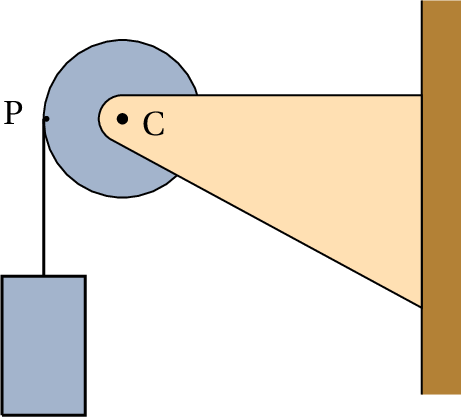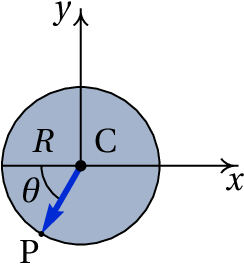Solution. Let us choose a system of Cartesian coordinates with origin at the center of the pulley's center, as shown in the figure. The figure also shows point P after the pulley has already rotated an angle from the initial position. The position vector for P at that moment is

The velocity of point P can be given in terms of the angular velocity of the pulley, which can be obtained from the velocity of the block. An expression for the velocity of the block can be derived integrating the equation

Since all points in the string have that same velocity and the points on the surface of the pulley follow the same motion as the string, is also the velocity for any point in the surface of the pulley and the angular velocity of the pulley is then . The angular velocity vector is perpendicular to the plane and since the rotation is in the anti-clockwise direction

The velocity vector of point P is equal to the cross product of the angular velocity vector and the position vector of point P

If the center of the pulley were moving, it would also be necessary to add its velocity to this last result. Note that the same result could have been obtained differentiating with respect to time, but it would have been necessary to find the expression for as a function of time.

The angular acceleration vector is the derivative of the angular velocity vector with respect to time

and the acceleration vector of point P is then

The expression for as a function of time is found by integrating the equation

substituting the values = 2, = 0.05 and = 9.8, in SI units, the velocity and acceleration at that time are found

## 3.6. Dependent translations and rotations

In a wheel rolling over a surface without sliding, the rotation angle and the displacement of the wheel's center are interrelated. Figure 3.11 shows a wheel of radius moving to the right, rolling over a surface without sliding.

If a point P on the surface of the wheel is initially in contact with the surface, after that point rotates an angle the center of the wheel moves a distance . The circular arc is equal to , because all the points in that arc were in contact with a point on the surface

(3.29)

Differentiating both sides of this equation, a relation between the velocity of the center C and the angular velocity is found

(3.30)

and differentiating one more time leads to the conclusion that the tangential acceleration of C is equal to the product of the radius and the angular acceleration

(3.31)

In the case of pulleys and strings, if the string does not slide over the surface of the pulley while this turns, the points on the surface of the pulley will have the same velocity as the string. The velocity of the points on the surface of the pulley relative to the center is equal to the velocity of those points, minus the velocity of the center of the pulley. That relative velocity divided by the radius of the pulley gives the angular velocity of the pulley

### Example 3.3

The radius of the static pulley in the system shown in the figure is 3 cm and the radius of the moving pulley is 5 cm. Find the velocity of the cart and the angular velocities of the pulleys, at a moment when the cylinder is falling with velocity equal to 1.5 m/s, assuming that the string does not slide over the pulleys.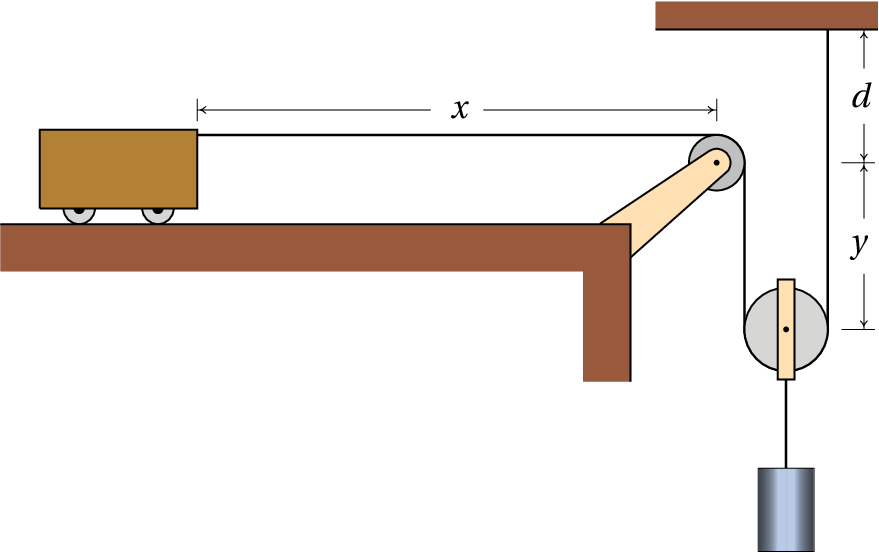Solution. This system has already been analyzed in Section 2.4 where it was shown that the velocity of the cart is always twice as great as the velocity of the cylinder. Therefore, the velocity of the cart at the instant considered is equal to 3 m/s.

In the static pulley, the velocity of the points on the surface are equal to the velocity of the cart, 3 m/s; hence, the angular velocity of that pulley is

The center of the moving pulley falls with the same velocity as the cylinder, 1.5 m/s. The point of the surface which is to the right of the center of the pulley is at rest; therefore, relative to the center of the pulley that point moves upward at 1.5 m/s. The point on the surface of the pulley which is to the left of the center moves downward with the same velocity as the cart, 3 m/s, so its velocity relative to the center of the pulley is 1.5 m/s downwards. The angular velocity of the moving pulley is then

The part of the string on the right of the moving pulley is static and thus could be regarded as a vertical surface over which the pulley is rolling down as a wheel moving over a surface. Therefore, the velocity of the center of the pulley, which is also equal to the velocity of the cylinder, is given by the angular velocity of the pulley times its radius. That's an alternative way to explain why the velocity of the cart is twice as great as the velocity of the cylinder, because the diameter of the pulley is twice as great as its radius.

### Example 3.4

The 2 meter bar shown in the figure is in contact with the horizontal floor in point A and with the vertical wall in point B. Initially, at , the distance is equal to  m and point A starts moving to the left with speed that depends on according to the following expression (SI units)

Meanwhile, point B moves down the wall. Find the angular velocity of the bar and the speed of point B, as functions of .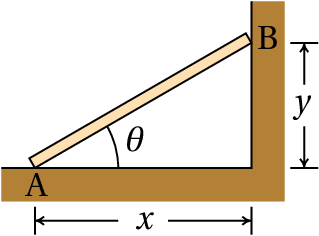Solution. This system has only one degree of freedom, which can be chosen as the distance . Since the length of the bar is 2, and are related to according to

The velocities of A and B are equal to the absolute value of the derivatives of and with respect to time and are obtained differentiating the two relations above

where is the angular velocity of the bar.

From the Pythagorean theorem, . The angular velocity is obtained substituting this expression into the equation for

and substituting this expression into the equation for leads to

Figure 3.12 shows the plot of the speed of B, from the initial instant when until the instant when the bar stops at the floor, when . That speed has a maximum value of around 9.7 cm/s, when the angle is around 57°.

## Questions

1. During the time interval 0 <   < 1, the velocity of an object as a function of time is . Knowing that the object follows a rectilinear trajectory, choose the correct expression for the acceleration from the following list.
2. The angular acceleration of an object with circular motion is constant and equal to  radian/s2. If the object starts moving from rest, in how many seconds will it complete 3 turns?
3. A point in an object follows a curved trajectory with constant velocity. Which of the following statements is correct?
1. The acceleration vector is perpendicular to the trajectory.
2. The magnitude of the acceleration vector is constant.
3. The acceleration vector is tangent to the trajectory.
4. The acceleration vector is constant.
5. The acceleration vector is null.
4. A projectile is thrown with initial velocity in a direction that makes an angle with the horizontal. Find the radius of curvature of the parabolic trajectory at the initial instant.
5. The rotation of a wheel of radius is being transmitted to another wheel of radius by a belt that moves with the two wheels, without sliding on their surfaces. Find the relation between the angular velocities and of the two wheels.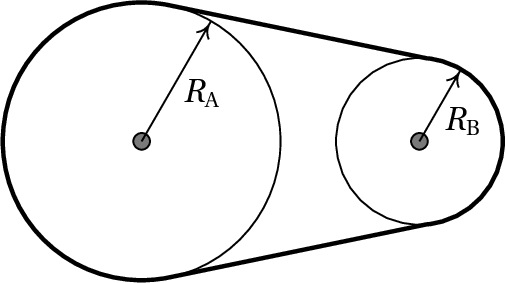## Problems

1. During the time interval the magnitudes of the velocity and the acceleration of a particle with three-dimensional motion are: and (SI units). Find the expressions, in that same time interval, for:
1. The tangential component of the acceleration.
2. The normal component of the acceleration.
2. A car driver enters a curve at 72 km/h, and slows down, making the speed decrease at a constant rate of 4.5 km/h each second. Make an estimate for the value of the radius of the curve using the scale shown in the figure. Find the acceleration of the car 4 seconds after the driver started to slow down.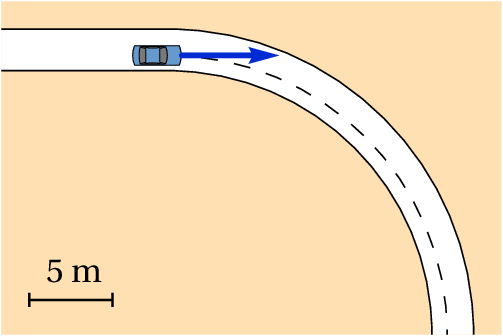3. The equation of the trajectory of an object is: (SI units and angles in radians). (a) Prove that the motion is circular and uniform. (b) Find the angular velocity of the object and its rotational period. (c) Find the position of the center of the circular trajectory.
4.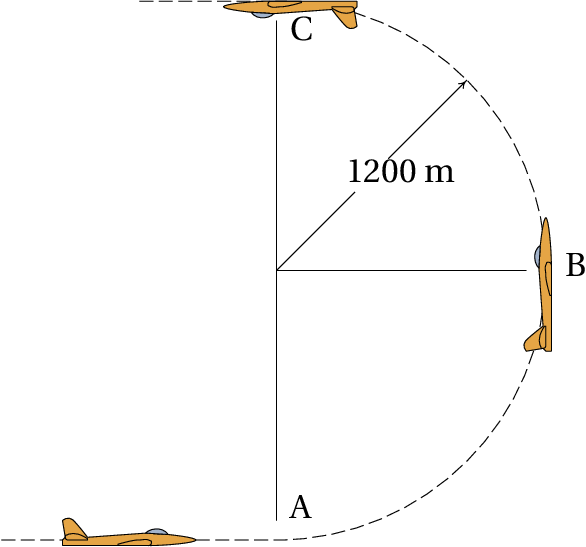An airplane race pilot performs a vertical loop following a semi-circumference of radius 1200 m. The airplane's velocity in point A, at the start of the loop, is 160 m/s and in point C, at the end of the loop it is 140 m/s. Find the acceleration in point B, at the middle of the loop, assuming that the tangential acceleration remains constant during the loop (note that it is also negative).
5. (a) Find the area of the triangle with vertices A, B and C with Cartesian coordinates A = (3, 5, 4), B = (−1,2,1) and C = (2,−2,2).
(b) Prove the law of sines, for a plane triangle with sides of length , and ,
where , and are the angles opposite to the sides , and respectively.
6.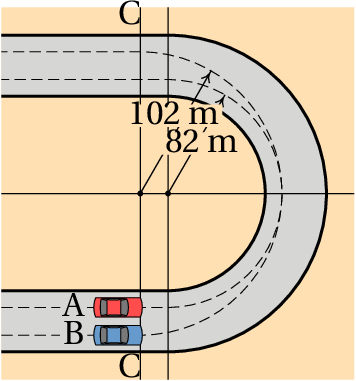Two cars A and B go through the curve shown in the figure following different paths. From a point on the line C, car B follows a semi-circumference of radius 102 m; until another point on line C. Car A moves from the line C following a straight line segment, it then follows a semi-circumference of radius 82 m and moves to another point on line C following another straight line segment. Both cars move at the highest speed that they can have without the tires sliding out of the circular path, which for the type of tires used means that the normal acceleration will have the maximum value of 0.8  , where is the acceleration of gravity. Find the times that both cars need to complete the curve, from the initial point on the line C, to the final point on the same line.
7.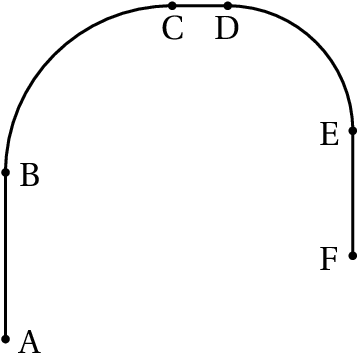A particle follows the path shown in the figure, starting from rest at A and then speeding up with constant acceleration until B. From B to E the speed of the particle remains constant at 10 m/s and at E the particle slows down, with constant acceleration, untill it stops at point F. The distance AB is 60 cm, CD is 20 cm and EF is 45 cm; arc BC has radius of 60 cm and arc DE has radius of 45 cm. Find:
1. The magnitude of the acceleration of the particle in each of the paths AB, BC, CD, DE and EF.
2. The total time of the motion from A to F and the average velocity in that motion.
8.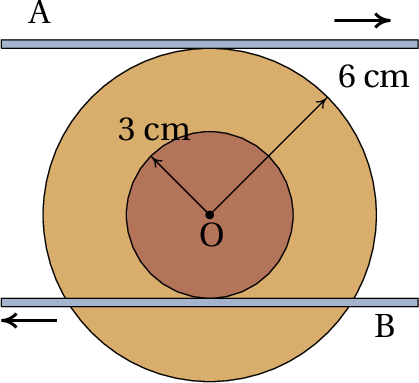A disk of radius 3 cm is glued to another disk of radius 6 cm, with a common axis, as shown in the figure. The horizontal bar A moves to the right at 10 m/s, keeping in contact with the bigger disk and without sliding on its surface. At the same time, the horizontal bar B moves to the left at 35 m/s, keeping in contact with the smaller disk and without sliding on its surface. Determine the direction of motion of the center O and find the velocity of O and the angular velocity of the disks.
9. A wheel of radius equal to 20 cm rolls without sliding over a plane horizontal surface, along the axis. At time the wheel's center is in and  cm and points P and Q in the wheel are in with and  cm. The velocity of the wheel's center remains constant and equal to 2 m/s. (a) Determine the time it takes the wheel to complete two turns. (b) Plot the trajectories of points P and Q in the interval of time corresponding to the two turns.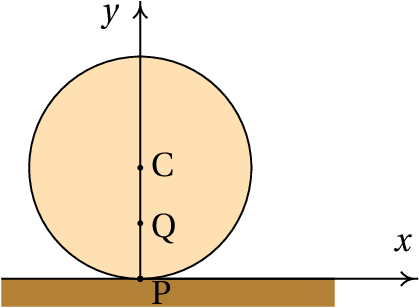10. A dumbbell has two disks with radius of 6 cm, connected by a cylindrical bar with radius of 4 cm. A long strip of paper is taped and rolled around the bar as shown in the figure. The dumbbell is then placed on a flat horizontal surface and the paper strip is pulled horizontally with a constant speed of 2.5 cm/s, making the dumbbell roll over the surface without sliding.
1. Find the angular velocity of the dumbbell.
2. Explain in which direction will move the point O in the axis of the bar and the disks and find its velocity.
3. How many centimeters of the paper strip will be wound or unwound per second?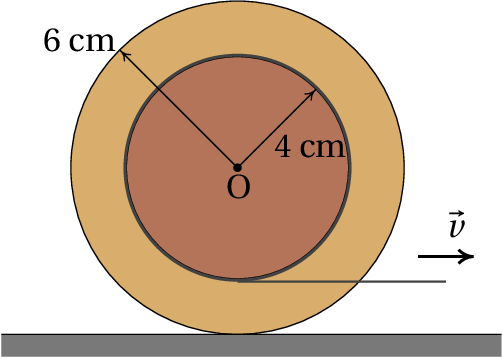11.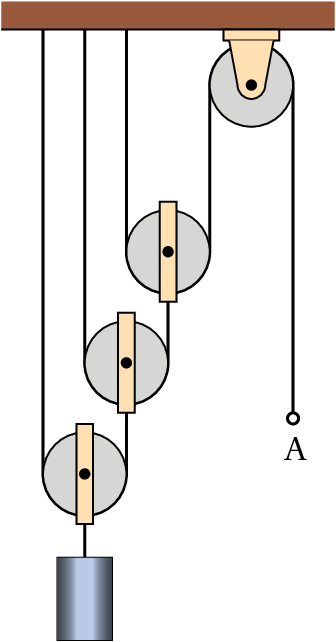All the pulleys in the configuration shown in the Figure have the same radius of 5 cm. Find the angular velocities of the four pulleys when the ring A is pulled down at 2 m/s.
12. The Figure shows a slider-crank mechanism used to convert circular motion to reciprocating motion (linear motion back and forth) or the other way around. The crank is the bar of length that rotates around a fixed axis through point O, and the bar of length used to link the crank to the sliding piston P is called the connecting rod. Choosing an axis along the direction in which the piston moves and through the points O and P, and if is the angle between the crank and the axis:
1. Show that the position of point P, as a as a function of , is given by
2. Find the relation between the angular velocity of the crank and the velocity of the piston.
3. The length of the connecting rod must be greater than . Make the plot of the velocity of the piston as a function of , in the case , and in the direction shown in the figure (SI units), and show that the speed of the piston is zero when equals 0 or 180°.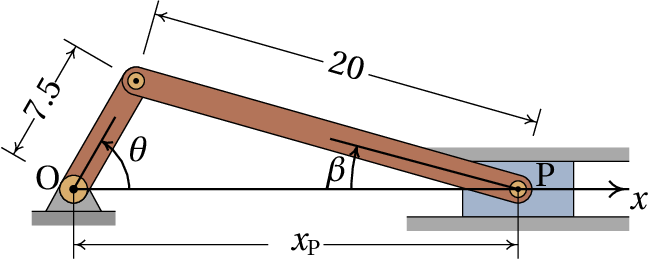Questions: 1. E. 2. B. 3. A. 4. E. 5. A.

Problems

1. (a)     (b)     (c)
2. With a radius of 16 m, the acceleration is approximately 14 m/s2
3. (a) The magnitude of the velocity vector is found to be and the components of the acceleration are found to be and . Therefore, the motion is uniform, because the velocity remains constant, and it is circular because the motion is on a plane and the radius of curvature, , is constant. (b)  rad/s, (seconds). (c) coordinates (4, 0).
4. 18.85 m/s2
5. (a) 14.79 (b) The three products ( ), ( ) and ( ) are all equal to twice the area of the triangle; equating each two of those three products, the three equations given are found.
6. 11.74 s for car A and 11.33 s for car B.
7. (a) 83.33 m/s2 in AB, 111.11 m/s2 in EF, 166.67 m/s2 in BC and 222.22 m/s2 in DE. (b) 0.395 s and 7.34 m/s.
8. Point O moves to the left, with velocity  m/s and the angular velocity of disks is  s−1.
9. (a) 1.26 s (b)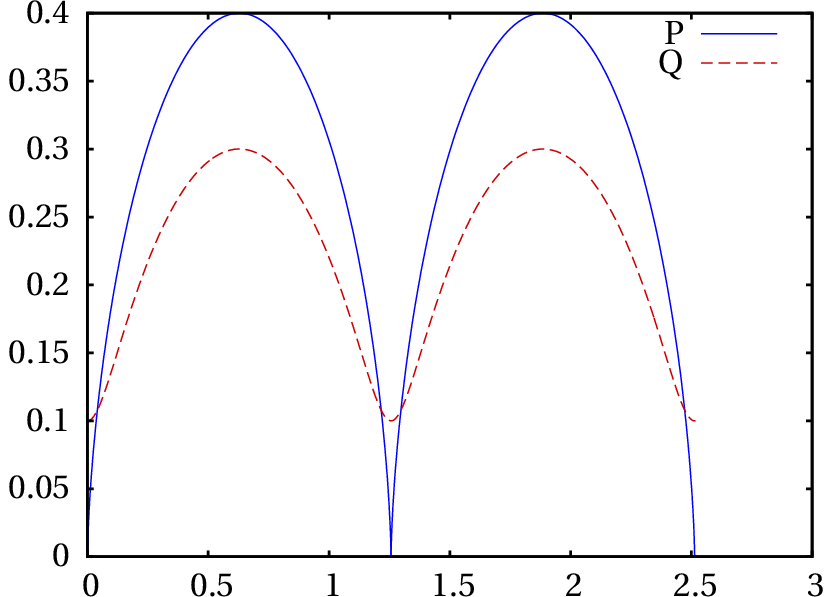10. (a) 1.25 s−1, in the clockwise direction. (b) To the right, with speed 7.5 cm/s. (c) 5 cm (the strip winds up more around the bar, because the bar is rotating clockwise).
11. From left to right, 5 s−1, 10 s−1, 20 s−1 and 40 s−1.
12. (b) (c) When equals 0 or 180°, and are both null and the expression for the velocity of Point P becomes 0.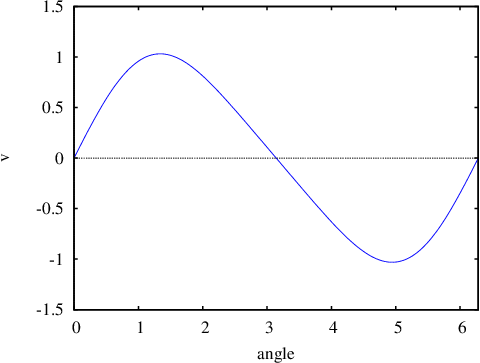Wrong

In a rectilinear motion the acceleration is equal to the tangential acceleration, which is the derivative of the velocity with respect to .

(click to continue)

Wrong

In a rectilinear motion the acceleration is equal to the tangential acceleration, which is the derivative of the velocity with respect to .

(click to continue)

Wrong

In a rectilinear motion the acceleration is equal to the tangential acceleration, which is the derivative of the velocity with respect to .

(click to continue)

Wrong

In a rectilinear motion the acceleration is equal to the tangential acceleration, which is the derivative of the velocity with respect to .

(click to continue)

Correct

In a rectilinear motion the acceleration is equal to the tangential acceleration, which is the derivative of the velocity with respect to .

(click to continue)

Wrong

The angular acceleration must be integrated with respect to time, to find the expression for the angular velocity and that expression must be integrated again to obtain the expression for the angle. Three turns mean an angle of .

(click to continue)

Correct

The angular acceleration must be integrated with respect to time, to find the expression for the angular velocity and that expression must be integrated again to obtain the expression for the angle. Three turns mean an angle of .

(click to continue)

Wrong

The angular acceleration must be integrated with respect to time, to find the expression for the angular velocity and that expression must be integrated again to obtain the expression for the angle. Three turns mean an angle of .

(click to continue)

Wrong

The angular acceleration must be integrated with respect to time, to find the expression for the angular velocity and that expression must be integrated again to obtain the expression for the angle. Three turns mean an angle of .

(click to continue)

Wrong

The angular acceleration must be integrated with respect to time, to find the expression for the angular velocity and that expression must be integrated again to obtain the expression for the angle. Three turns mean an angle of .

(click to continue)

Correct

The acceleration vector has only normal component because the tangential acceleration (derivative of the velocity with respect to time) is zero.

(click to continue)

Wrong

The normal acceleration, related to the change of direction of the velocity, could have any value; therefore, the magnitude of the acceleration can change.

(click to continue)

Wrong

The acceleration vector can't be tangent to the trajectory, because the tangential component of the acceleration (derivative of the velocity with respect to time) is zero.

(click to continue)

Wrong

There are curvilinear motions with constant acceleration vector. An example is the parabolic motion of a projectile. However, since the normal acceleration has different directions at different points in the trajectory, there must be also a variable tangential acceleration that compensates those changes. In this case, that cannot happen because the tangential acceleration is zero.

(click to continue)

Wrong

If the acceleration vector was null, the trajectory should be a straight line, but in this case it is curved.

(click to continue)

Wrong

The radius of curvature is equal to the square of the velocity, divided by the normal acceleration. The normal acceleration is obtained projecting the acceleration of gravity vector along the direction of the velocity vector.

(click to continue)

Wrong

The radius of curvature is equal to the square of the velocity, divided by the normal acceleration. The normal acceleration is obtained projecting the acceleration of gravity vector along the direction of the velocity vector.

(click to continue)

Wrong

The radius of curvature is equal to the square of the velocity, divided by the normal acceleration. The normal acceleration is obtained projecting the acceleration of gravity vector along the direction of the velocity vector.

(click to continue)

Wrong

The radius of curvature is equal to the square of the velocity, divided by the normal acceleration. The normal acceleration is obtained projecting the acceleration of gravity vector along the direction of the velocity vector.

Correct

The radius of curvature is equal to the square of the velocity, divided by the normal acceleration. The normal acceleration is obtained projecting the acceleration of gravity vector along the direction of the velocity vector.

(click to continue)

Correct

The speed of any point in the belt is the same and hence, the speeds of the points on the surfaces of the two wheels must be the same.

(click to continue)

Wrong

The speed of any point in the belt is the same and hence, the speeds of the points on the surfaces of the two wheels must be the same.

(click to continue)

Wrong

The speed of any point in the belt is the same and hence, the speeds of the points on the surfaces of the two wheels must be the same.

(click to continue)

Wrong

The speed of any point in the belt is the same and hence, the speeds of the points on the surfaces of the two wheels must be the same.

(click to continue)

Wrong

The speed of any point in the belt is the same and hence, the speeds of the points on the surfaces of the two wheels must be the same.

(click to continue)